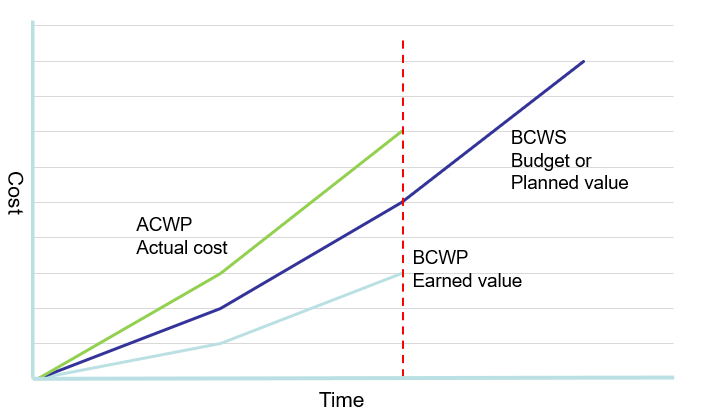# What does Budgeted Cost of Work Scheduled mean in Project Management?

Budgeted Cost of Work Scheduled
The Budgeted Cost of Work Scheduled is the sum of the budget for all planned work scheduled within a given time frame. This measurement often indicates the cumulative budget up to a certain point in time.BCWS is a crucial component of project management. It is vital for tracking project performance, providing a baseline for comparing actual costs and work progress.

## How to calculate the BCWS

To calculate BCWS, one must first identify all the tasks planned for a specific period within the project. Next, allocate a budget for each task, considering factors such as labor, materials, and other resources required to complete the task. Finally, the sum of all these individual task budgets represents the BCWS for the given period.
Here is the formula:
BCWS = % Complete (Planned) x Project Budget.

## A worked example of calculating the BCWS

Let's consider a construction project with a total budget of \$500,000. The project completion is due in 5 months. We want to calculate the Budgeted Cost of Work Scheduled (BCWS) for the first three months.

First, we must determine the planned percentage of work completed each month. Let's assume the following:
Month 1: 20% of the work is due.
Month 2: 30% of the work is due.
Month 3: 25% of the work is due.

Now, we can calculate the BCWS for each month:
Month 1 BCWS: (20% x \$500,000) = \$100,000 Month 2 BCWS: (30% x \$500,000) = \$150,000 Month 3 BCWS: (25% x \$500,000) = \$125,000

To find the cumulative BCWS for the first three months, we add the BCWS values for each month:
Cumulative BCWS (Months 1-3) = \$100,000 + \$150,000 + \$125,000 = \$375,000

So, the Budgeted Cost of Work Scheduled for the first three months of the construction project is \$375,000.

## How to use BCWS

Project managers use BCWS to monitor project performance and identify potential budget overruns or schedule delays. By comparing BCWS with actual costs and work progress, managers can detect discrepancies and take corrective actions to ensure the project stays on track. This comparison often uses metrics like Cost Performance Index (CPI) and Schedule Performance Index (SPI).In short, BCWS is an essential aspect of project management that helps in budget allocation, performance monitoring, and schedule adherence. By understanding the budgeted costs of scheduled work, project managers can make informed decisions to ensure the project's success.
See all project management words